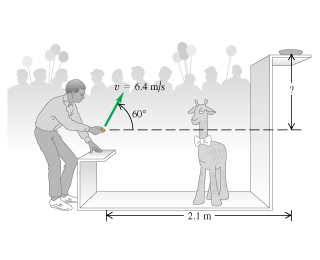# Problem: In a carnival booth, you win a stuffed giraffe if you toss a quarter into a small dish. The dish is on a shelf above the point where the quarter leaves your hand and is a horizontal distance of 2.1 m from this point (the figure ). If you toss the coin with a velocity of 6.4  m/s at an angle of 60 (Pages 75 - 82) above the horizontal, the coin lands in the dish. You can ignore air resistance.You may want to review  (Pages 75 - 82) .For related problemsolving tips and strategies, you may want to view a Video Tutor Solution of Height and range of a projectile i: a batted baseball.A. What is the height of the shelf above the point where the quarter leaves your hand?B. What is the vertical component of the velocity of the quarter just before it lands in the dish?

###### FREE Expert Solution

We're going to consider the following kinematic equations:

A.

R = vxt = vcosθt

Solving for and calculating time:

t = R/vcosθ = 2.1/(6.4)cos(60°) = 21/32 s

86% (182 ratings)###### Problem Details
In a carnival booth, you win a stuffed giraffe if you toss a quarter into a small dish. The dish is on a shelf above the point where the quarter leaves your hand and is a horizontal distance of 2.1 m from this point (the figure). If you toss the coin with a velocity of 6.4  m/s at an angle of 60 (Pages 75 - 82) above the horizontal, the coin lands in the dish. You can ignore air resistance.

You may want to review .

For related problemsolving tips and strategies, you may want to view a Video Tutor Solution of Height and range of a projectile i: a batted baseball.

A. What is the height of the shelf above the point where the quarter leaves your hand?

B. What is the vertical component of the velocity of the quarter just before it lands in the dish?